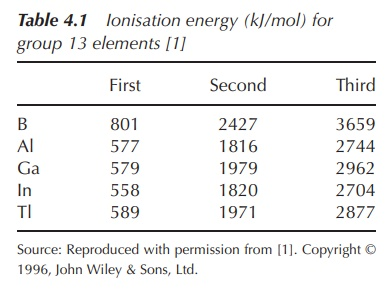# General chemistry of group 13 elements

| Home | | Inorganic Pharmaceutical Chemistry |

## Chapter: Essentials of Inorganic Chemistry : The Boron Group - Group 13

Group 13 elements are characterised by having three electrons in their valence shell. Therefore, all elements form the stable cation M3+.

General chemistry of group 13 elements

Group 13 elements are characterised by having three electrons in their valence shell. Therefore, all elements form the stable cation M3+. Most elements (with the exception of B) form additionally the singly positively charged ion M+, which is indeed the more stable oxidation state for Tl.

Boron and aluminium occur only with oxidation number +3 in their compounds, and with a few exceptions their compounds are best described as ionic. The electronic configuration shows three electrons outside a noble gas configuration, two in an s shell and one in a p shell. The outermost p electron is easy to remove as it is furthest from the nucleus and well shielded from the effective nuclear charge. The next two s electrons are also relatively easy to remove. Removal of any further electrons disturbs a filled quantum shell and is therefore difficult. This is reflected in the ionisation energies (Table 4.1).The main sources of B are the two minerals borax (Na2[B4O5(OH)4]8H2O) and kernite (Na2[B4O5(OH)4]), which are generally used as components in many detergents or cosmetics. Al occurs widely on earth, and it is the most abundant metal and the third most abundant element in the earth’s crust.

Aluminosilicates, such as clays, micas, feldspar, together with bauxite, are the main sources of Al. Ga, In and Tl occur in traces as their sulfides.

## Extraction

Boron (B) can be extracted from borax by converting the latter to boric acid (Equation 4.1) and subsequently to the corresponding oxide (Equation 4.2). Boron of low quality can then be obtained by the reduction of boron oxide with Mg, followed by several steps of washing with bases and acids.

Na2[B4O5(OH)4] 8H2O + H2SO4 4B(OH)3 + Na2SO4 + 5H2O           (4.1)

2B(OH)3 B2O3 + 3H2O               (4.2)

Al is extracted from ores such as bauxite or cryolite in the so-called Bayer process. Bauxite contains mainly a mixture of aluminium oxides with Fe2O3, SiO2 and TiO2 as impurities. In the Bayer process, hot aqueous NaOH is added to the crude ore under pressure and aluminium hydroxide will go into solution. This will result in the separation of Fe2O3. The solution is cooled down and seeded with Al2O33H2O in order to precipitate Al(OH)3. Pure Al can be produced by electrolysis of molten Al2O3 (melting point 2345 K), with Al being obtained at the cathode (Figure 4.2).The main source of Ga is bauxite, but it can also be obtained from the residues from the Zn processing industry. It can be found in the zinc sulfide ore sphalerite. Tl can be obtained as by-product of the processing of Cu and Zn ores. The demand for In and Tl is rather low.

## 1. Reactivity

B is chemically unreactive except at high temperatures. Al is a highly reactive metal, which is readily oxi-dised in air to Al2O3. This oxide coating is resistant to acids but is moderately soluble in alkalis. Al itself dissolves in diluted mineral acids (Equation 4.3) and can react with strong alkalis, the product being the tetrahydroxoaluminate ion [Al(OH)4] and H2 (Equation 4.4).

Al + 3H2SO4(aq) Al(SO4)3 + 3H2                                                    (4.3)

2Al + 2MOH + 6H2O 2M[Al(OH)4] + 3H2                                                    (4.4)

Aluminium can be used to reduce metal oxides, the most famous example being the thermit process. Al reacts violently with iron(Ill) oxide to produce iron in this highly exothermic process, where Fe is obtained in its liquid form (Equation 4.5).

2Al(s) + Fe2O3(s) 2Fe(l) + Al2O3(s)

Ga, In and Tl dissolve in most acids, and as a result the salts of Ga(III), In(III) and Tl(I) are obtained, whereas only Ga reacts with aqueous alkali with the production of H2.

## 2. Oxides/hydroxides: amphoteric compounds

Boron oxide (B2O3) is an acidic oxide and an insoluble white solid with a very high boiling point (over 2000 K) as a result of its extended covalently bonded network structure. Aluminium oxide (Al2O3, Equations 4.6 and 4.7) as well as aluminium hydroxide (Al(OH)3, Equations 4.8 and 4.9) are amphoteric compounds.

Amphoteric compounds are substances that can react either as an acid or as a base.

Al2O3 + 3H2O + 2(OH) 2[Al(OH)4]                                                   (4.6)

Al2O3 + 3H2O + 6[H3O]+ 2[Al(H2O)6]3+                                                   (4.7)

Al(OH)3 can neutralise a base and therefore act as an acid (Equation 4.8); it can also neutralise an acid and act as a base (Equation 4.9).

Al(OH)3 + NaOH NaAl(OH)4                                                   (4.8)

Al(OH)3 + 3HCl AlCl3 + 3H2O                                                   (4.9)

## 3. Halides

The most important halide of boron is the colourless gas boron trifluoride (BF3). Aluminium chloride (AlCl3) is a volatile solid which sublimes at 458 K. The vapour formed on sublimation consists of an equilibrium mixture of monomers (AlCl3) and dimers (Al2Cl6). It is used to prepare the powerful and versatile reducing agent lithium tetrahydridoaluminate (LiAlH4).

Both boron trichloride (BCl3) and aluminium trichloride (AlCl3) act as Lewis acids to a wide range of electron-pair donors, and this has led to their widespread use as catalysts. In the important Friedel–Crafts acylation, AlCl3 is used as a strong Lewis acid catalyst in order to achieve the acylation of an aromatic ring.

A Lewis acid is defined as a compound that can accept electrons pairs with the formation of a coordinate covalent bond. Any type of electrophile can be a Lewis acid. In contrast, Brønsted–Lowry acids are com-pounds that transfer a hydrogen ion (H+) and they are the more commonly known type of acids. Analogous definitions apply for a Lewis base (electron donator) and a Brønsted–Lowry base (H+ acceptor).